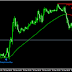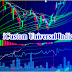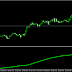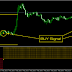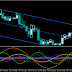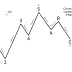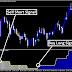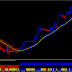Post Title Index and Item -->

Forex 4-Hour Stochastic EMA Trend Trading Strategy relies heavily on catching the trend. You may have success using this strategy on as low as the one hour chart or as high as the daily chart; however, I’ve had most success trading it on the four-hour chart.

Forex 4-Hour Stochastic EMA Trend Trading Strategy consists of four indicators, which are:-
1.  5 Period Exponential Moving Average (closed)
2.  15 Period Exponential Moving Average (closed)
3.  50 Period Exponential Moving Average (closed)
4.  Stochastic indicator K=13 D=5 Smooth=5 (13,5,5) 80/20 Levels
Your chart should look like this:-There are only two, yet very important rules to follow when trading this strategy.

1. The K% line on the stochastic indicator MUST be above the D% line when taking a BUY & the K% line MUST be below the D% line when taking a SELL.

2. Both the 5 & 15 EMA MUST cross the 50 EMA going upwards to create a BUY signal OR downwards for a SELL signal.

Let’s take a look at some examples:-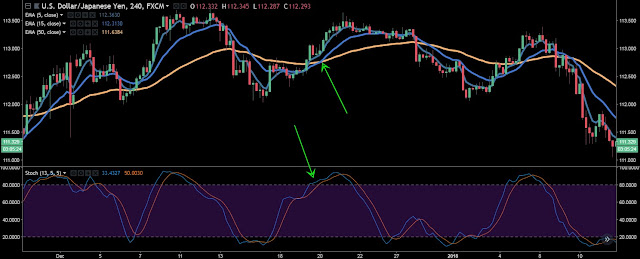In this example, we can see the K% is above the D% (as shown by the bottom green arrow). We can also see the 5 & 15 EMA have crossed the 50 EMA (as indicated by the upper green arrow). Once both the 5 & 15 EMA’s have crossed the 50 EMA going upwards, we would have taken a BUY.

Next example:In this example, we can see the K% is below D% (as shown by the bottom red arrow). We can also see the 5 & 15 EMA have crossed the 50 EMA (as indicated by the upper red arrow). Once both the 5 & 15 EMAs have crossed the 50 EMA going downwards we would have taken a SELL.

As you can see, in both of these examples, we would have caught the trend and made some major pips.
This concludes the Forex 4-Hour Stochastic EMA Trend Trading Strategy